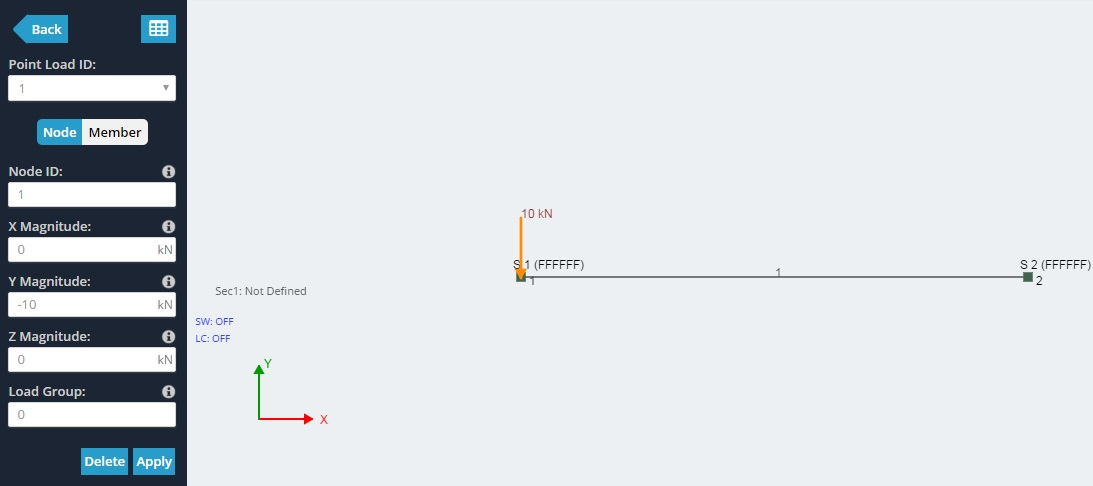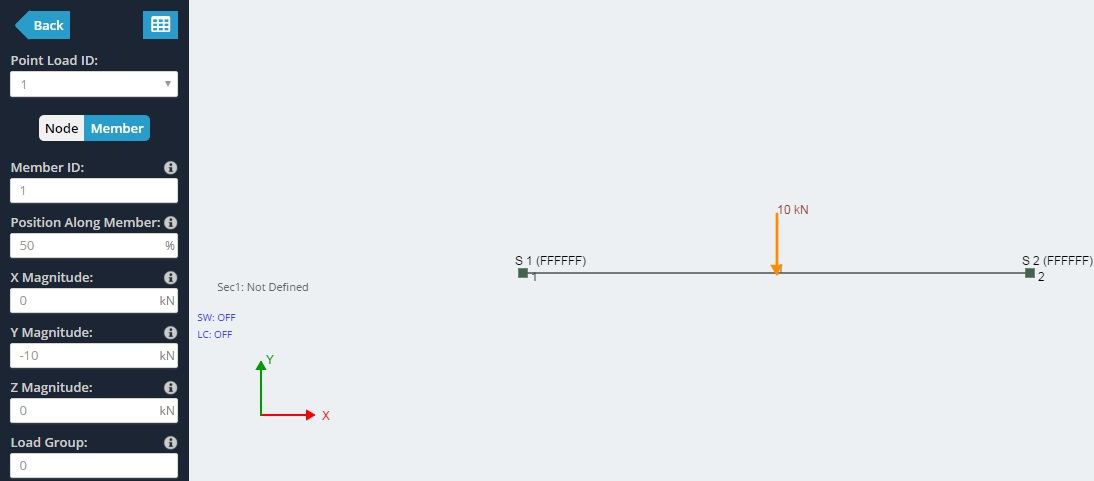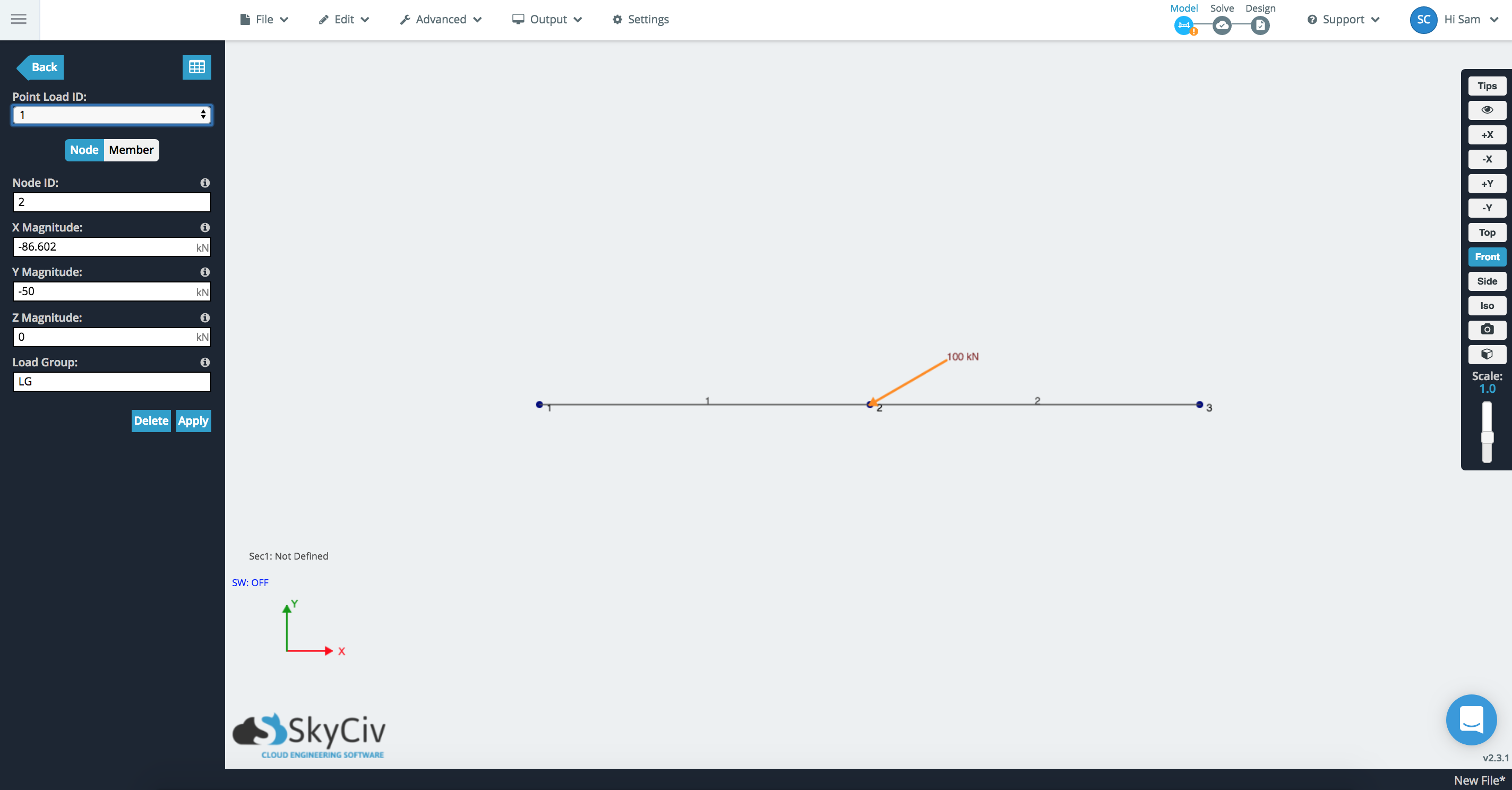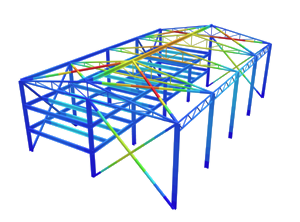SkyCiv Documentation

Your guide to SkyCiv software - tutorials, how-to guides and technical articles

1. Home
2. SkyCiv Structural 3D

Point loads are forces that can be applied at a node or at a point along with a member. In both cases, the location, magnitude, and sign direction need to be specified. Forces that are applied at an angle to the node or member can be specified by providing the X, Y, Z components of the force.

## Point Loads applied to Nodes

To apply a point load on a node, simply specify values for:

• Point Load ID – The numerical ID used to identify each point load.
• Node ID – The node where the point load is applied.
• X Magnitude – The magnitude of the force in the global X-direction. Use negatives to specify the negative X direction.
• Y Magnitude – The magnitude of the force in the global Y-direction. Use negatives to specify the negative Y direction.
• Z Magnitude – The magnitude of the force in the global Z-direction. Use negatives to specify the negative Z direction.## Point Loads applied to Members

To apply a point load on a member, simply specify values for:

• Member ID – The member where the point load is applied.
• Position Along the Member – The % position (from node A t B) along the member where the force is to be applied.
• X Magnitude – The magnitude of the force in the global X-direction. Use negatives to specify the negative X direction.
• Y Magnitude – The magnitude of the force in the global Y-direction. Use negatives to specify the negative Y direction.
• Z Magnitude – The magnitude of the force in the global Z-direction. Use negatives to specify the negative Z direction.Here’s a short example of how to apply a point load at an angle. For example, say you want to apply a -100kN load at a 30-degree angle to the XY plane. Seeing as there are infinite planes and directions we can apply this point load to, we need to use the magnitude to specify the force at an angle.

We need to break the force into two parts: the X magnitude and Y magnitude, we would do this as follows:

Xmag = -100cos(30) = -86.602 kN
Ymag = -100sin(30) = -50 kN

Combined this will create a 100 kN force at a 30* angle:

### Quick Tip

You can enter equations and formulas (for instance, -100cos(30)) into SkyCiv input boxes, and we’ll actually evaluate and enter the value (-86.602) to save you time. Use this for quick calculations, and to save you entering long and tedious numbers manually. Some other examples are:

• 3.14*2
• 6.32e-10
• 2.2^3
• 3+4